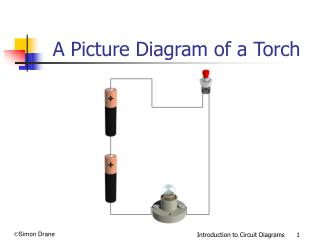DownloadDownload PresentationA Picture Diagram of a Torch

# A Picture Diagram of a Torch

Download Presentation## A Picture Diagram of a Torch

- - - - - - - - - - - - - - - - - - - - - - - - - - - E N D - - - - - - - - - - - - - - - - - - - - - - - - - - -
##### Presentation Transcript

1. A Picture Diagram of a Torch Introduction to Circuit Diagrams

2. British Standard Circuit Symbols Battery Switch Bulb Introduction to Circuit Diagrams

3. A Circuit Diagram of a Torch Introduction to Circuit Diagrams

4. Basic Electronic Components Bulb Switch Battery Buzzer Resistor L.E.D. Motor Diode Capacitor Introduction to Circuit Diagrams

5. Exercise 1 Circuit Diagrams Bulb Switch Battery Buzzer Resistor L.E.D. Motor Diode Capacitor Introduction to Circuit Diagrams

6. Exercise 2 Circuit Drawing Using British Standard Symbols draw a circuit diagram of the above circuit. Introduction to Circuit Diagrams

7. Circuit Diagram for Exercise 2 Introduction to Circuit Diagrams

8. Exercise 3 1 Diode 3 Resistor L.E.D 4 2 Capacitor Identify the electronic components in the following circuit. Introduction to Circuit Diagrams

9. Exercise 4 Using British Standard Symbols draw a circuit diagram of the above circuit. Introduction to Circuit Diagrams

10. Circuit Diagram for Exercise 4 Introduction to Circuit Diagrams